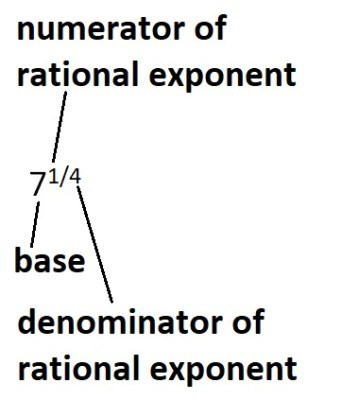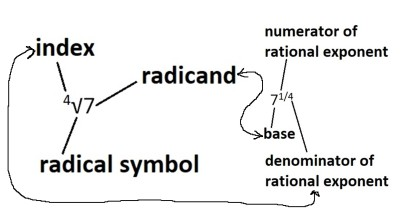# Radicals & Rational Exponents (3 Key Concepts)

Radicals come up often in algebra, geometry, calculus, and other subjects in math.  Converting between radicals and rational exponents is helpful in simplifying expressions and solving equations.

So, what do you need to know about radicals & rational exponents?  A radical tells you to take a root of a number (called the radicand). The index “n” (to the top left of the radical symbol) tells you which root to take (n = 2 for a square root, n = 3 for a cube root, etc.) A rational exponent is a power a/b, where b is the index of the corresponding radical.

Of course, the most common radical is one with no index listed.  In this case, the index is understood to be n = 2, which tells us to take a square root.

In this article, we’ll talk about radicals, rational exponents, and how they are related.  We’ll also look at some examples to make the concepts clear.

Let’s get started.

Remember that a radical expression has three parts:

• The radical symbol (this tells us that we are taking a root of some number).
• The radicand (this is the number under the radical symbol – we are taking the nth root of the radicand).
• The index (this tells us which root we are taking – an index of n means we are taking the nth root of the radicand).

Similarly, a term with a rational exponent has three parts:

• The base (this is the number that we are raising to a power that is the numerator of a rational exponent).
• The numerator of the rational exponent (this is the power that we are raising the base to)
• The denominator of the rational exponent (this is the root we are taking).This image shows the three parts of a term with a rational exponent for the fourth root of 7: the base (R = 7), and the rational exponent (1/4) with a numerator of 1 and a denominator of 4.

Note that the following parts of radical expressions and rational exponents correspond:

• The radicand in the radical expression and the base of the rational exponent term are the same.
• The index of the radical and the denominator of the rational exponent are the same.

You can see this illustrated in the image below:We can see that the radicand and the base (R = 7) match up, as do the index and denominator of the rational exponent (n = 4).

The following expression shows us how radical expressions and rational exponents are related:

• n√(Rm) = Rm/n

Note that we can also write the left side of the equation above as:

• (R1/n)m = Rm/n

In the special case when m = 1, we get the simplified equation:

• n√(R) = R1/n

So, “the nth root of R” is the same as “R raised to the power of 1/n”.

Let’s look at some examples.

#### Example 1: Radical Expressions & Rational Exponents

• √5

Whenever there is no index written for a radical, we assume an index of n = 2.  Another way to write this radical is

• 2√5

Using the equation from before, we can convert this radical expression to a term with a rational exponent:

• n√(R) = R1/n
• 2√(5) = 51/2  [here, the radicand is R = 5, and the index is n = 2 for a square root]

So, √5 and 51/2 mean the same thing, and they are the same number (about 2.236).

#### Example 2: Radical Expressions & Rational Exponents

• 3√7

Here, we can see that we have an index of n = 3 (to the top left of the radical symbol) and a radicand of R = 7 under the radical symbol.

Using the equation from before, we can convert this radical expression to a term with a rational exponent:

• n√(R) = R1/n
• 3√(7) = 71/3  [here, the radicand is R = 7, and the index is n = 3 for a cube root]

So, 3√7 and 71/3 mean the same thing, and they are the same number (about 1.913).

#### Example 3: Radical Expressions & Rational Exponents

• 4√(23)

Here, we can see that we have an index of n = 4 (to the top left of the radical symbol) and a radicand of R = 23 under the radical symbol.

Using the equation from before, we can convert this radical expression to a term with a rational exponent:

• n√(Rm) = Rm/n
• 4√(23) = 23/4  [here, the radicand is R = 23, and the index is n = 4 for a fourth root]

So, 4√(23) and 23/4 mean the same thing, and they are the same number (about  1.682).

Note that we could also write this number as 4√(8), since 23 = 8.  We could also write this as 81/4.

#### Example 4: Radical Expressions & Rational Exponents

• 5√(1024)

Here, we can see that we have an index of n = 5 (to the top left of the radical symbol) and a radicand of R = 1024 under the radical symbol.

Using the equation from before, we can convert this radical expression to a term with a rational exponent:

• n√(R) = R1/n
• 5√(1024) = 10241/5  [here, the radicand is R = 1024, and the index is n = 5 for a fifth root]

So, 5√1024 and 10241/5 mean the same thing, and they are the same number (4).

How did we get 4?  Well, 1024  is a power of 2 – specifically: 1024 = 210.  Using this in the rational exponent form gives us:

• 10241/5
• =(210)1/5
• =210*(1/5)  [since (xa)b = xa*b, by the rules of exponents]
• =22  [since 10/5 = 2]
• =4

### What Exponent Is Square Root?

A square root implies an exponent of ½, or an index of n = 2 for the corresponding radical.  As mentioned earlier, if a radical has no index listed, then we assume an index of 2 (a square root).

For example, √5 = 51/2, which means that the square root of 5 is the same as 5 to the power of ½ (a rational exponent of ½).

### What Happens To An Exponent In A Square Root (Radical)?

If we have an exponent inside a square root (radical), we get a new exponent by dividing the exponent by the index of the radical (by the rules of exponents).

Here is the proof: if we have the nth root of xm, we get:

• n√(xm)  [m is the exponent inside the radical, and n is the index of the radical]
• =(xm)1/n  [since n√A = A1/n for any number A; here, A = xm]
• =xm*(1/n)  [since (xa)b = xa*b, by the rules of exponents]
• =xm/n  [the exponent inside the radical, m, is divided by the index n]

#### Example 1: An Exponent In A Radical

If we take the 7th root of 35, we get:

• 7√(35)
• =(35)1/7
• =35*(1/7)
• =35/7

#### Example 2: An Exponent In A Radical

• 9√(34/5)
• =(34/5)1/9
• =3(4/5)*(1/9)
• =34*1/5*9
• =34/45

### What Happens To An Exponent Outside A Square Root (Radical)?

If we have an exponent outside a square root (radical), we get a new exponent by dividing the exponent by the index of the radical (by the rules of exponents).

Here is the proof: if we have the mth power of the nth root of x, we get:

• (n√x)m  [m is the exponent outside the radical, and n is the index of the radical]
• =(x1/n)m  [since n√x = x1/n for any number A]
• =x(1/n)*m  [since (xa)b = xa*b, by the rules of exponents]
• =xm/n  [the exponent inside the radical, m, is divided by the index, n]

### How To Solve Square Roots (Radicals) Raised To A Power

To solve a square root (or any radical) raised to a power, we just use the rule we found above.  Here are some examples.

#### Example 1: An Exponent Outside A Radical

If we take the 6th power of the 5th root of 7, we get:

• (5√7)6
• =(71/5)6
• =7(1/5)*6
• =76/5

#### Example 2: An Exponent Outside A Radical

If we take the 9th power of the 4th root of 52, we get:

• (4√(52))9
• =((52)1/4)9  [since a 4th root means a power of ¼]
• =((52*(1/4))9  [since (xa)b = xa*b, by the rules of exponents]
• =((52/4))9
• =(51/2)9  [2/4 = ½ after we reduce fractions]
• =5(1/2)*9  [since (xa)b = xa*b, by the rules of exponents]
• =59/2

## Conclusion

Now you know a little more about radicals, rational exponents, and how the two ideas are connected.  You also know how to solve problems that involve these concepts.